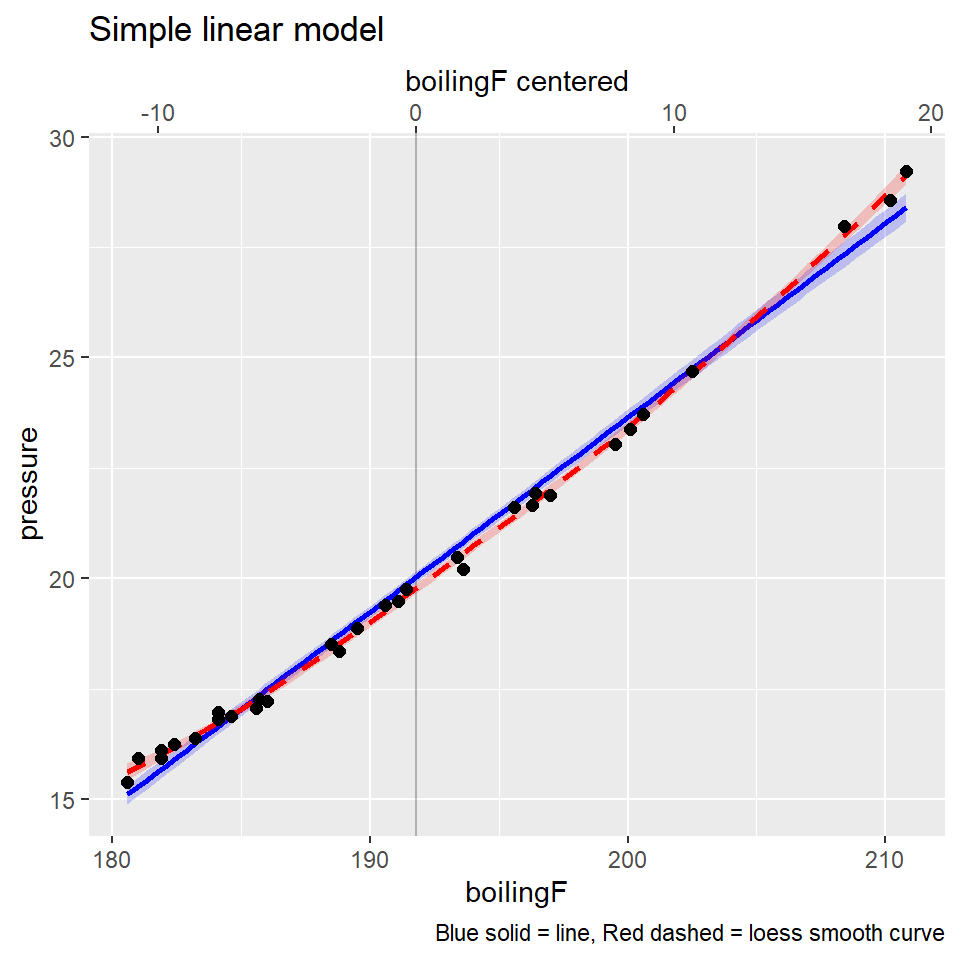# 1 Hooker’s Himalayian boiling point altitude data

Dr. Joseph Hooker collected the following data in the 1840s on the boiling point of water and the atmospheric pressure at 31 locations in the Himalayas. Boiling point is measured in degrees Fahrenheit. The pressure is recorded in inches of mercury, adjusted for the difference between the ambient air temperature when he took the measurements and a standard temperature.

The goal was to develop a model to predict the atmospheric pressure from the boiling point.

Historical note: Hooker really wanted to estimate altitude above sea level from measurements of the boiling point of water. He knew that the altitude could be determined from the atmospheric pressure, measured with a barometer, with lower pressures corresponding to higher altitudes. His interest in the above modelling problem was motivated by the difficulty of transporting the fragile barometers of the 1840s. Measuring the boiling point would give travelers a quick way to estimate elevation, using the known relationship between elevation and barometric pressure, and the above model relating pressure to boiling point.

library(erikmisc)
library(tidyverse)

dat_boil <-
, skip = 2
) %>%
mutate(
boilingF_cen = boilingF - mean(boilingF)
)

# x-variable mean for centering
dat_boil$boilingF %>% mean()  191.7871 str(dat_boil) spec_tbl_df [31 x 3] (S3: spec_tbl_df/tbl_df/tbl/data.frame)$ boilingF    : num [1:31] 211 210 208 202 201 ...
$pressure : num [1:31] 29.2 28.6 28 24.7 23.7 ...$ boilingF_cen: num [1:31] 19.01 18.41 16.61 10.71 8.81 ...
- attr(*, "spec")=
.. cols(
..   boilingF = col_double(),
..   pressure = col_double()
.. )
- attr(*, "problems")=<externalptr> 

## 1.1(2 p) Plot the data.

Using ggplot, try to implement these features in a plot. Overlay both a straight-line regression line in blue (geom_smooth(method = lm, col = "blue", ...)), as well as a loess smooth (default) dashed line in red (geom_smooth(method = loess, col = "red", linetype = 2, ...)). Using alpha=1/5 will make the confidence bands more transparent. Also, if you plot the points last, they’ll lie on top of the lines.

Describe the key features of this plot.

### 1.1.1 Solution

I’ll give you this first plot to help get started, in particular to illustrate a nice use of the caption and the annotation of a second x-axis for the centered version of the boilingF variable.

library(ggplot2)
p <- ggplot(dat_boil, aes(x = boilingF, y = pressure))
p <- p + scale_x_continuous(sec.axis = sec_axis(~ . - mean(dat_boil$boilingF), name = "boilingF centered")) p <- p + geom_vline(xintercept = mean(dat_boil$boilingF), alpha = 1/4)
p <- p + geom_smooth(method = lm, se = TRUE, col = "blue", fill = "blue", alpha = 1/5)
p <- p + geom_smooth(method = loess, se = TRUE, col = "red", fill = "red", linetype = 2, alpha = 1/5)
p <- p + geom_point(size = 2)
p <- p + labs(title = "Simple linear model"
, caption = "Blue solid = line, Red dashed = loess smooth curve"
)
print(p)## 1.2(3 p) Fit a simple linear regression, assess assumptions.

Fit a simple linear regression model for predicting pressure from boiling point. Provide output for examining residuals, outliers, and influential cases.

Looking at the plots, are there any indications that the mean pressure is not linearly related to boiling point? Are there any observations that appear to be highly influencing the fit of this model? Are there certain points or regions of the data where the model does not appear to fit well? Discuss.

Which, if any, of the standard linear regression model assumptions appears to be violated in this analysis? If you believe that some of the assumptions are violated, does it appear that deleting one or two points would dramatically improve the fit? Would you use this model for predicting pressure from boiling point? Discuss and carry out any needed analysis to support your position.

## 1.3(1 p) Interpret $$R^2$$

Interpret $$R^2$$ in the previous simple linear regression model.

## 1.4(2 p) A better model.

Decide whether transformation, or a polynomial model in boiling point, is needed to adequately summarize the relationship between pressure and boiling point. If so, perform a complete analysis of the data on this scale (that is, check for influential observations, outliers, non-normality, etc.).

## 1.5(2 p) Final model.

Regardless of which scale you choose for the analysis, provide an equation to predict pressure from boiling point. Write a short summary, pointing out any limitations of your analysis.

### 1.5.1 Solution

Assuming you called your linear model object lm_p_b1, then the equation with code below will place the intercept and slope in the equation. Just add an r before each of the signif(...) inline code chunks to make the numbers appear. Then use this example to write your final model here.
$\widehat{\textrm{pressure}} =  signif(coef(lm_p_b1), 3) +  signif(coef(lm_p_b1), 3) \textrm{ boilF}$